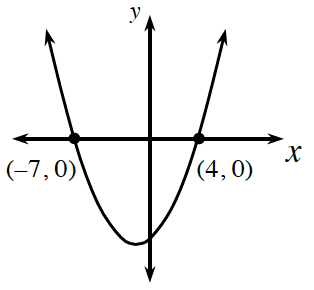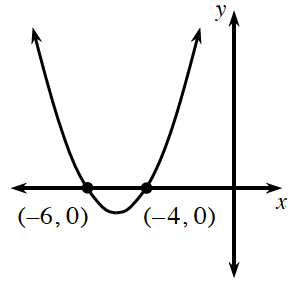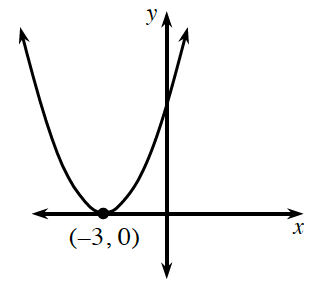### Home > CCAA8 > Chapter 11 Unit 12 > Lesson CCA: 11.1.1 > Problem11-13

11-13.

Based on each graph, give a possible equation for the parabola.

1.A quadratic equation forms a parabola when graphed. When $x$ equals $−7$ or $4$, $y$ equals $0$. This means that $−7$ and $4$ are roots of the equation. If you know the roots, can you find the factors for a possible equation?

The factors for a possible equation are
$(x+7)\ \text{and}\ (x−4)$.

$y=(x+7)(x−4)$

1.See the hint for part (a).

1.See the hint for part (a). Because the parabola intersects the $x$-axis at only one point, its equation has a single factor, which is squared.

Use the eTool below to help you write and check a possible equation for each parabola.
Click on the link at right for the full eTool version: CCA 11-13 HW eTool